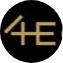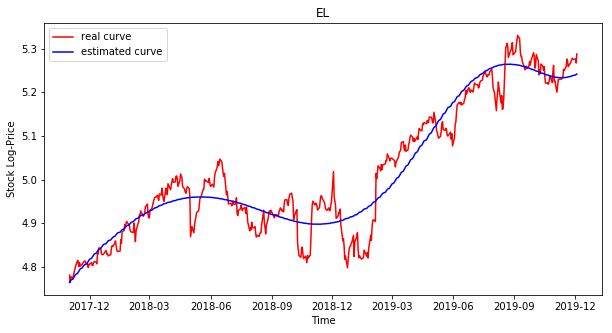Search
•4E Capital AG

# Volatility and Bubble Forecast

Updated: Feb 11, 2020

GARCH(1,1) model:

We are currently working on an analysis of volatility prediction on the S&P 500 using the GARCH(1,1) model. This model is an indicator of the possible behavior of the volatility in the next days and weeks.The method:

The idea is to produce estimates of the current and future levels of volatility based on historical measures of the long-run average variance rate, logarithmic returns, and past volatility values. In order to do that, we calculate the magnitude of these three components that influences future volatility. In other words, we compute the optimal values of the weights of the long-run variance rate (gamma), the logarithmic returns (alpha) and the past volatility values (beta) of the following equation.GARCH(1,1) Model

The results:

On November 6th, 2019, we forecasted the volatility of the S&P 500 for the next 3 weeks. Our results predicted that the volatility would increase for that period with a significant confidence interval. These results were verified in January 2020.Forecast S&P 500 Volatility

Bubble Prediction Model:

We are working on an analysis of bubble prediction based on the LPPL model (designed by Prof. Sornette ETH Zurich).The method:

In a nutshell, this method is a combination of the maximal value of a given stock (A), the positive feedback during the creation of the bubble (B summand), and the periodic oscillations (C summad) increasing at the end of the bubble that represents the panic of traders before the crash. With the price data of a given stock, we aim to estimate the 7 parameters describing the behavior of the underlying. With the goal to estimate the key parameter of the LPPL model (t_c) which represents the time of the change of regime.LPPL Model

The results:

On December 5th, 2019, we analyzed different indices and stocks for the possibility of a bubble. We found that 2 stocks within the S&P 500 fulfill the characteristics of a possible bubble based on the LPPL model. This quantitative indicator is a hint for us to pay closer attention to those stocks closer to their possible change of regime. The estimation of the change of regime depends heavily on the correct guess of the beginning of the bubble which is not trivial and is subject to future research.

See All